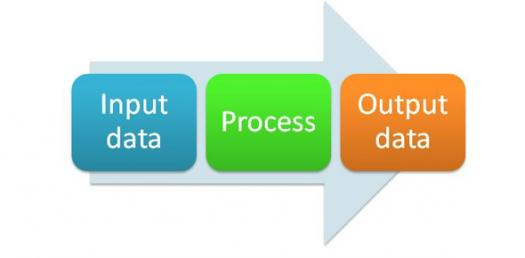# Preparation Of Data For Input

10 Questions | Total Attempts: 139SettingsStudy the topic Preparation of Data for Input before you try this test.

• 1.
A number used to determine the accuracy of a code is called a check digit
• A.

True

• B.

False

• 2.
Check digits completely verify the accuracy of a number and so eliminate all possible errors
• A.

True

• B.

False

• 3.
All valid data is by necessity correct
• A.

True

• B.

False

• 4.
Validation checks always ensure that the entered data is reasonable and complete.
• A.

True

• B.

False

• 5.
Range checks, control digits and check data are all examples of validation tests.
• A.

True

• B.

False

• 6.
Transcription errors can occur when data is being copied from one medium to another.
• A.

True

• B.

False

• 7.
All transposition errors are transcription errors.
• A.

True

• B.

False

• 8.
Data verification is one type of data validation
• A.

True

• B.

False

• 9.
Data verification involves comparing two sets of data in order to ensure that the entered data is correct.
• A.

True

• B.

False

• 10.
Using modulus 11 the check digit for the number 472 is 3
• A.

True

• B.

False

Related TopicsBack to top